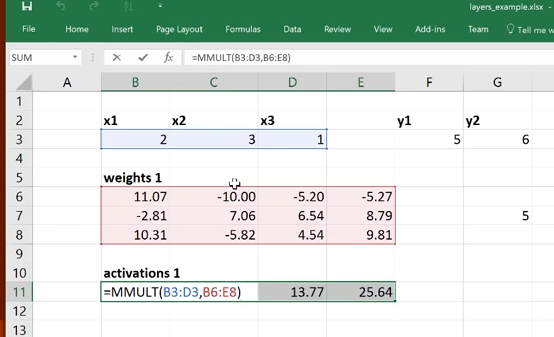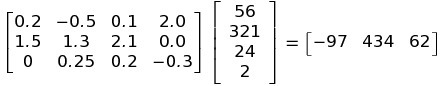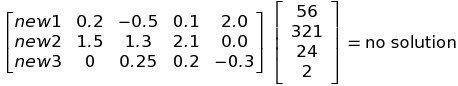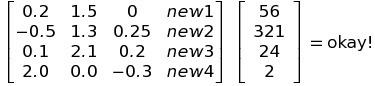# Contradictory views of the matrix multiplication in a layer?

I was looking at how to set up the matrix multiplication in deep learning. Playing around with the dimensionality in general is confusing, especially given Keras helpful-but-seemingly-lasse-faire approach to specifying output dimensions.

Anway, when watching the video of the second lesson, there’s a part where Jeremy showed the mock up of some data travelling through a DL network. Here at exactly this spot, (pause it!) we can see the formula typed in the cell that is responsible for doing the matrix multiplication:A few things jump out at me, and please someone chime in if this is agreeable or now:

• The structure of this operation, MMULT, is [x] . |W| = [y]. What I’m trying to say is that the data (pixels or whatnot) is on the LEFT.
• With the data on the left, we aren’t restricted to having only 3 columns in the Weight matrix. If you keep playing the video, you can see how he’s choosing different numbers of columns in W at any iteration. The way he has it set up, he’s free to choose W of any size he wants, any time.
• If the data were on the RIGHT, then we would be constricted to only 3 columns in the Weight matrix.
• I’m suspecting there is a big valuable thing going on here – perhaps this is how we are able to reduce the dimensionality of the raw data, while increasing the semantic information in the network as we progress through the layers.

Everything I just wrote is all just conjecture, because I don’t know.

But the real confusion kicks in when I check out the Neural Networks for Visual Recognition reading from the Stanford class. Here, the linear operation is shown to be the opposite as we just saw in Jeremy’s example, that is, | W | . [x] = |y|

How are we supposed to set up the matrix multiplication? Am I right to suspect Jeremy’s way has some significance to it? Any why is the Stanford example different?
Thanks,
Group #16

2 Likes

Splendid question - and hopefully will be the start of an interesting conversation.

Firstly - the boring bit: in Excel I had my input and activation vectors as rows, whereas in the Stanford class (and more commonly) they’re considered columns. That’s why they are multiplied in different orders (but the outcome is identical, except for being transposed.)

Next up - note that the spreadsheet can be analyzed by methods other than pausing the video; you can download it! http://www.platform.ai/files/xl/layers_example.xlsx

Most importantly - let’s discuss the dimensionality of the weight matrices. The number of rows of each of the weight matrices in the spreadsheets is clearly fixed - it has to equal the number of items in the input vector that it is being multiplied by. But the number of columns can be anything you like! More specifically: the number of columns you put in the weight matrix in the spreadsheet determines the number of activations it creates.

So in keras, when you create a dense layer, you write:

``````Dense(n)
``````

The ‘n’ here is the number of activations that you want you Dense layer to create. And therefore it’s defining the dimensionality of the weight matrix for that layer. You don’t need to include the other dimension of the weight matrix, because, as we noted above, it’s entirely defined by the size of the layer’s input. So keras is kind enough to calculate that for you.[Important note: the first parameter of Convolution2D() is doing exactly the same thing - it defines how many filters that layer creates, and therefore defines the dimensionality of the weight matrix for that convolutional layer.]

I understand the perspective from the DL’s layers, and the layer’s parameters in Keras. All that feels way more firm in my head now. Thanks!

But specifically regarding the matrix multiplication only, I must be screwing up something very simple.

If I introduce another column to W as shown in the Stanford cat picture, that matrix cannot be solved. There are more variables than solutions and the system is indeterminate. Correct?

W would have to be transposed for the solution to be found. But I don’t see any transposing anywhere.

Let’s stick to the Excel spreadsheet for this discussion, so we are both in agreement about what we mean by rows and columns…

We don’t “solve” anything. We’re only doing a matrix multiplication. If we add another column to the weight matrix in the spreadsheet, that creates an output vector with one additional element.

The contents of the weight matrix are initialized randomly. They are then updated using SGD.

Is that clear now? If not, maybe try opening the spreadsheet and playing around with it - try creating the formulae for the matrix multiplication by hand, rather than using MMULT(), if you want to be really sure you understand the details.

I am just trying to figure out the matrix operations in general.

We don’t “solve” anything.

By solve, I am referring to the structure of the operation.

Let’s stick to the Excel spreadsheet for this discussion, so we are both in agreement about what we mean by rows and columns…

Would it be okay to just take a look at the Stanford example? Excel doesn’t explicity have the notion of a vector (that I know of!) so maybe sticking with the more explicity-shaped Stanford example would be more clear.

(Sorry to be a pain, and I’m sure this is a forehead slapper and I’ll feel pretty silly afterwards…)

Say we have this system, again, borrowed from the Stanford page (but i got rid of the bias, to make is simpler):

W*X = yEverything looks great.

But when I add a new column on the Weight matrix, this doesn’t work:Another way to look at it is the good ole dimensionality double check trick:

3 X 4 X 4 X1 ==> 1 X 3
but
3 X 5 X 4 X 1 ==> no solution.

The only way this system can avoid degeneracy is to throw in a transpose:

W^t*X = y…but this looks really nonsensical.

So I don’t see how the image on the Stanford page is going to work, if we want to extend the W matrix by adding columns.

I must have something set up wrong?

Matt

Because everything in the Stanford example is transposed, compared to the Excel example, you extend the Stanford example by adding rows, not columns. Then that will work nicely, right?..That is very frustrating! I spent all day on this – why didn’t the Stanford folks call the matrix

W^t

instead of W?? Grrr!!!In retrospect, the matrix MUST be transposed – if not, each training example would have consisted of only one channel, since it’s one color per row.

You should probably blame me, not Stanford - although neither is wrong, just different. The way around they have it is more common for math notation; I just used the orientation that is more convenient in a spreadsheet.

PS: In general, it’s helpful to remember that: a.T.dot(b.T) = b.dot(a).T

2 Likes

I love this thread and had exactly the same problem. If I’m understanding this correctly the question is, why transpose our input vector (row in dataset representing a single observation) into column form in order to make matrix multiplication work given the following formula?

`f(xi,W,b) = Wxi + b`

When we could accomplish the same thing by rewriting the formula as:

`f(xi,W,b) = xiW + b`

And creating a Weights (W) matrix with the same number of rows as our observation has columns. We maintain the flexibility of expanding our Weights matrix, but in this case our lever is the # of columns, not rows.

So far it sounds like this is entirely arbitrary, just the convention mathematicians established. But perhaps there is some convenience or simplicity gained by writing it the conventional way?

It’s entirely arbitrary